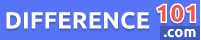Scalar

Scalar refers to a mathematical quantity that has only magnitude and no direction. In simpler terms, scalar is a physical quantity that can be measured and expressed through a single numerical value, without any additional information about where it came from or in which direction it is moving. Scalar quantities are represented by a simple number, unit of measurement, and dimensional analysis. Examples of scalar quantities include distance, time, mass, speed, temperature, and energy. Understanding scalars is essential to many fields of science, including physics, chemistry, and engineering, as it lays the foundation for the analysis of complex mathematical concepts and calculations.